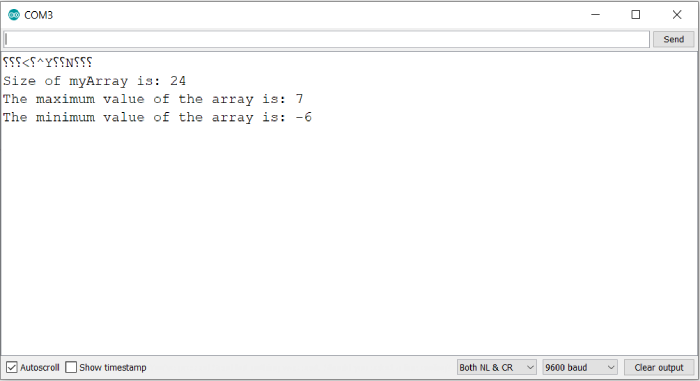# Get max and min values of an array in Arduino

In order to get the max/ min values of an array in Arduino, we can run a simple for loop. Two implementations are shown below. One uses the max() and min() functions of Arduino, and the other uses the > and < operators.

The max and min functions have the following syntax: max(a,b) and min(a,b), and they return the max and min values out of a and b respectively.

## Implementation 1 − Using > and < operators

void setup() {
// put your setup code here, to run once:
Serial.begin(9600);
Serial.println();
int myArray = {1, 5, -6, 4, -2, 7};

int maxVal = myArray;
int minVal = myArray;
Serial.print("Size of myArray is: "); Serial.println(sizeof(myArray));

for (int i = 0; i < (sizeof(myArray) / sizeof(myArray)); i++) {
if (myArray[i] > maxVal) {
maxVal = myArray[i];
}
if (myArray[i] < minVal) {
minVal = myArray[i];
}
}
Serial.print("The maximum value of the array is: "); Serial.println(maxVal);
Serial.print("The minimum value of the array is: "); Serial.println(minVal);
}

void loop() {
// put your main code here, to run repeatedly:
}

## Implementation 2 − Using max and min

void setup() {
// put your setup code here, to run once:
Serial.begin(9600);
Serial.println();
int myArray = {1, 5, -6, 4, -2, 7};

int maxVal = myArray;
int minVal = myArray;
Serial.print("Size of myArray is: "); Serial.println(sizeof(myArray));

for (int i = 0; i < (sizeof(myArray) / sizeof(myArray)); i++) {
maxVal = max(myArray[i],maxVal);
minVal = min(myArray[i],minVal);
}

Serial.print("The maximum value of the array is: ");
Serial.println(maxVal);
Serial.print("The minimum value of the array is: ");
Serial.println(minVal);
}

void loop() {
// put your main code here, to run repeatedly:
}

## Output

The Serial Monitor output in both the cases is −As you can see, the sizeof() function is returning the total number of bytes and not the number of elements in the array (I’m using a board which stores int in 4 bytes). Therefore, within the for loop, the condition has been kept as −

i < (sizeof(myArray) / sizeof(myArray))

Updated on: 29-May-2021

6K+ Views